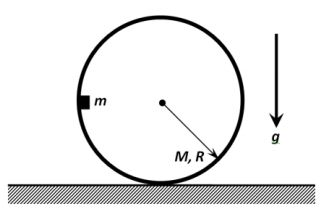# IPhO 2014 Theoretical Problem #1A small puck of mass $m$ is carefully placed onto the inner surface of the thin hollow thin cylinder of mass $M$ and of radius $R$. Initially, the cylinder rests on the horizontal plane and the puck is located at the height $R$ above the plane as shown in the figure on the left. Find the interaction force $F$ between the puck and the cylinder at the moment when the puck passes the lowest point of its trajectory. Assume that the friction between the puck and the inner surface of the cylinder is absent, and the cylinder moves on the plane without slipping. The free fall acceleration is $g$.

$F = pmg( q + \frac m {M} )$, where $p,q$ are integers. Find $p + q$.

×